StatLect

Predictive model

In this lecture we introduce the concept of a predictive model, which lies at the heart of machine learning (ML).Observed input-output mapping

To begin with, we observe some outputsand the corresponding input vectorsthat may help to predict the outputs before they are observed.

Examples:

•is the total amount of purchases made by a customer while visiting an online shop;are some characteristics of the landing page that was first seen by the customer;

•is inflation observed in monthandis a vector of macro-economic variables known before;

•is 1 if firmdefaults within a year and 0 otherwise;is a vector of firm's characteristics that may help to predict the default;

•is a measure of economic activity in province;is a vector of pixel values from a satellite image of the province.

Note: the subscriptused to index the observations is not necessarily time.

Prediction

We use the observed inputs and outputs to build a predictive model, that is, a functionthat takes new inputsas arguments and returns the predictions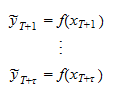of previously unseen outputs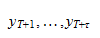Some machine learning jargon

Before diving into predictive modelling, let us learn some machine learning jargon.

Supervised vs unsupervised learning

The problem of learning an input-output mapping is called a supervised learning problem.

The data used for learning is called labelled data and the outputsare called labels or targets.

Basically, in a supervised learning problem, the task is to learn the conditional distribution of the outputs given the inputs.

On the contrary, in an unsupervised learning problem, there are no labels and the task is to learn something about the unconditional distribution of.

The typical example are photos of cats and dogs:is a vector of pixel values; in supervised learning, you have labels(1 if dog, 0 if cat); in unsupervised learning, you have no labels, but you typically do something like clusteringin the hope that the algorithm autonomously separates cats from dogs.

Regression vs classification

A supervised learning problem is called:

• a classification problem if the output variableis discrete / categorical (e.g., cat vs dog);

• a regression problem if the output variableis continuous (e.g., income earned).

Features

The inputs are often called features and the vectoris called a feature vector.

Training

The act of using data to find the best predictive model (e.g., by optimizing the parameters of a parametric model) is called model training.

Loss function

How do we assess the quality of a predictive model?

How do we compare predicted outputswith observed outputs?

We do these things by specifying a loss function, which is always required in a machine learning problem.

A loss function quantifies the losses that we incur when we make inaccurate predictions.

Examples:

• Squared Error (SE):• Absolute Error (AE):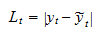• Log-loss (or cross-entropy):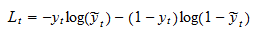whenis binary (i.e., it can take only two values, either 0 or 1); the multivariate generalization is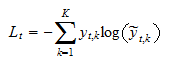whenis amultinoulli vector (i.e., we have a categorical variable that can take onlyvalues; when it takes the-th, thenand all the other entries of the vectorare zero).

Risk

Ideally, the best predictive models is the one having the smallest statistical risk (or expected loss)where the expected value is with respect to the joint distribution ofand.

Empirical risk

Since the true joint distribution ofandis usually unknown, the risk is approximated by the empirical riskwhereis a set of input-output pairs used for calculating the empirical risk andis its cardinality (the number of input-output pairs contained in).

Thus, the empirical risk is the sample average of the losses over a set of observed data. This is the reason why we sometimes call it average loss.

How to chooseis one of the most important decisions in machine learning and we will discuss it at length.

For specific choices of the loss function, empirical risk has names that are well-known to statisticians:

• if the loss is the Squared Error, then the empirical risk is the Mean Squared Error (MSE), and its square root is the Root Mean Squared Error (RMSE);

• if the loss is the Absolute Error, then the empirical risk is the Mean Absolute Error (MAE);

• if the loss is the Cross-Entropy, it can easily be proved that the empirical risk is equal to the negative average log-likelihood.

Empirical risk minimization

The criterion generally followed in machine learning is that of empirical risk minimization:

• if we are setting the parameters of a model, we choose the parameters that minimize the empirical risk;

• if we are choosing the best model in a set of models, we pick the one that has the lowest empirical risk.

Statistically speaking, it is a sound criterion because empirical risk minimizers are extremum estimators.